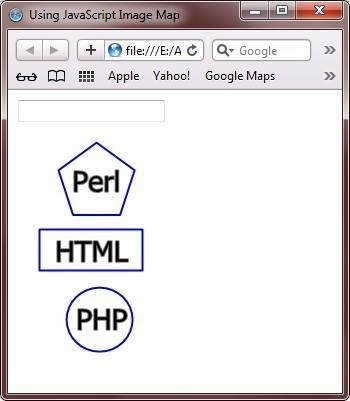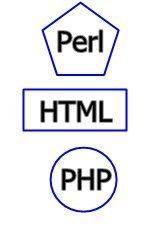# JavaScript Image Map

An image map in JavaScript is an image on a web page that provides various links to navigate to other web pages or some sections of the same web page. You can say those various links as hotspots.

In an image map in JavaScript to provide hotspot link, you can use any shape such as rectangle, circle, or polygon.

If you want to create a rectangular image map, then you need two different co-ordinates, such as top right and bottom left. If you want to create a circular image map, then you need centre co-ordinate. If you want to create a polygon image map, then you need different number of co-ordinates. And last, if you want to create a pentagon shape image map, then you need five co-ordinates.

Use MAP element to define image maps. Every image map has a unique name. Therefore, the name attribute is required in the MAP element. You can add `usemap` attribute to the `IMG` element to associate an image map with an image.

## JavaScript Image Map Example

Here is an example demonstrates image map in JavaScript:

```<html>
<head>
<title>Using JavaScript Image Map</title>
<script type="text/javascript">
function show_image_map_value(name)
{
document.image_map_form.stage.value = name
}
</script>
</head>
<body>

<form name="image_map_form">
<input type="text" name="stage" size="20" />
</form>

<img src="image-map.jpg" alt="javascript image map" border="0" usemap="#tutorial_links"/>

<map name="tutorial_links">
<area shape="poly" coords="80,1,118,30,102,75,56,75,41,32" href="/perl/index.htm" alt="Perl Tutorial"
onMouseOver="show_image_map_value('Go to Perl Tutorial')" onMouseOut="show_image_map_value('')"/>

<area shape="rect" coords="25,87,128,129" href="/html/index.htm" alt="HTML Tutorial"
onMouseOver="show_image_map_value('Go to HTML Tutorial')" onMouseOut="show_image_map_value('')"/>

<area shape="circle" coords="83,178,32" href="/php/index.htm" alt="PHP Tutorial"
onMouseOver="show_image_map_value('Go to PHP Tutorial')" onMouseOut="show_image_map_value('')"/>
</map>

</body>
</html>```

Here is the sample output produced by the above JavaScript image map example code:You can click on the Perl, HTML, or PHP to go to the tutorial page.

Here is the live demo output produced by the above image map example code in JavaScript:Tools
Calculator

Quick Links
Signup - Login - Give Online Test